# Triangle free strongly regular graphs

 Importance: High ✭✭✭
 Author(s):
 Subject: Graph Theory » Algebraic Graph Theory
 Keywords: strongly regular triangle free
 Posted by: mdevos on: May 28th, 2007
Problem   Is there an eighth triangle free strongly regular graph?

A regular graphis strongly regular if there exist integers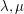so that every pair of adjacent vertices have exactly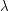common neighbors, and every pair of nonadjacent vertices have exactlycommon neighbors. To eliminate degeneracies, we shall further assume that. Ifis-regular and, then we say thatis astrongly regular graph.

There are exactly seven triangle-free strongly regular graphs known: The five cycle, the Petersen Graph, The Clebsch Graph, the Hoffman-Singleton Graph, The Gewirtz Graph, the Higman-Sims Graph, and a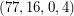strongly regular subgraph of the Higman-Sims graph. Every Moore Graph of diameter 2 is a triangle-free strongly regular graph, so if there is a 57-regular Moore Graph of diameter 2, this would add another to the list.

See Andries Brouwer's graph descriptions for more on these graphs.

## Bibliography

[G] C. D. Godsil, Problems in Algebraic Combinatorics, Electronic Journal of Combinatorics, Volume 2, F1

* indicates original appearance(s) of problem.

### Higman-Sims Graph

In fact, all (seven) known primitive triangle-free strongly regular graphs are actual *subgraphs* of the Higman-Sims graph (which btw was first constructed by Dale Mesner). A Moore graph of degree 57 would of course break this mold.

I hate math..Lol _________________________________________________________________________________________ toll free number

### The complement of $2 K_n$

The complement of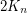is triangle free srg with parameters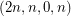. Probably this infinite case should be excluded from the conjecture.

### If the number of the

If the number of the vertices are even, we can determine regular triangle free graphs up to half the number of vertices of any degree. However, this does not hold true if the vertices numbers are odd. Some of the examples of even vertices graphs are Petersen graph (10 vertices), Heawood graph (14 vertices), Clebsch graph (16 vertices), Pappus graph (18 vertices) and odd vertices graph includes Schläfli graph (27 vertices), Perkel graph (57 vertices). 800 numbers